# Semi-conductor components: diode model

## Diode: behavior

The behavior of a diode, as well as the diode operation, can be deduced from the current-voltage characteristic:

ID = f (UD).

This characteristic is represented on the figure on the right.## Linear approximation

It is often easier to consider the diode in an approximate manner. Hence the simple models in the table below are proposed.(1)(2)(3)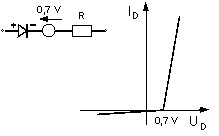(4)

## The model Ron-Roff of Flux

The simplest model proposed in Flux for a diode is called the Ron-Roff model. It relates to model 2 in the table above.

The necessary quantities to define the ID = f (UD) characteristic by this model are:

• the on state resistance Ron ; the value of this resistance should be low enough, generally of the order of 0.1 Ω
• the off state resistance Roff ; the value of this resistance must be large enough, generally of the order of 10,000 Ω

To represent a diode using model 4, it is necessary to juxtapose a Flux component diode and a voltage source.

## Exponential approximation

A more accurate model of the current-voltage characteristic of a diode can be carried out using the following exponential function: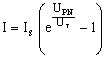where:

• UPN is the voltage at the terminals of the PN junction
• UT is the thermodynamic voltage or temperature voltage*
• IS is the saturation current
Note:

* The temperature voltage can be computed using the following formula: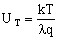where:

• k is the Boltzmann constant (k = 1,38 × 1023 J/K)
• T is the absolute temperature of the component
• λ is an empiric constant (0,5 ≤ λ ≤ 1)
• q is the elementary charge of the electron (q = 1,6 × 1019 C)

## Equivalent diagram and the U(I) characteristic

If we consider the equivalent diagram of the diode represented in the figure below, the voltage UD at the diode terminals is given by the sum:

UD = URserial + UJunctionPN

The voltage-current characteristic UD = f (ID) is expressed by the relation: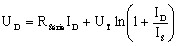## The exponential model of Flux

The model proposed for the diode in Flux, called the Exponential model, corresponds to the model presented previously.

Because the value of the temperature voltage UT is not easy to get, the value of the threshold voltage or of the knee voltage VF0 is used in Flux 3D for the exponential model.

The relation linking UT,V F0 and IS. is the following: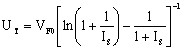The parameters necessary for the definition of a diode using this model are represented in the figure below.These are:

• the knee forward voltage: VF0 (in V)

• the extrinsic resistance: RS (in Ω) (or bulk resistance)

• the saturation current: IS (in A)

Typical values for these three parameters are proposed by default : VF0 = 0,7V ; RS = 0,1Ω ; IS = 106 A

Note: The reverse breakdown voltage is not used in Flux.